Definitions of Square Dance Calls and Concepts

Index -->  Plus  |  A1  |  A2  |  C1  |  C2  |  C3A  |  C3B  |  C4  |  NOL  |
Definitions (Text Only) -->  Plus  |  A1  |  A2  |  C1  |  C2  |  C3A  |  C3B  |  C4  |  NOL  |
 Find call:

1/4 (or 3/4) The Deucey -- [C3A]
(Cliff Rosser \$B\$H(B Ed Foote 1973)

\$BJ?9T\$J(B Wave \$B\$+\$i!%(B

1/4 The Deucey: Arm Turn 1/4 \$B\$r\$7\$F(B, Centers \$B\$,(B Cast Off 1/4 \$B\$r\$9\$k4V\$K(B, Lead End \$B\$O(B Circulate \$B\$r(B, Trailing End \$B\$O(B 1/2 Circulate \$B\$r\$7\$F(B, Center Star \$B\$,(B turn 1/4 \$B\$r\$7(B, \$B2q\$C\$??M\$,(B (Center Wave) Cast Off 1/4 \$B\$r\$7\$F(B, lonesome Center \$B\$O(B move up (Phantom Hourglass Circulate) \$B\$r\$7(B Wave \$B\$N(B End \$B\$K\$J\$j\$^\$9!%J?9T\$J(B Wave \$B\$G=*\$o\$j\$^\$9!%(B

3/4 The Deucey: \$B3F!9\$N(B 1/4 turn \$B\$,(B 3/4 turn \$B\$K\$J\$k0J30\$O(B 1/4 The Deucey \$B\$HF1\$8\$G\$9!%(B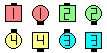1/4 The Deucey\$B\$NA0(B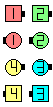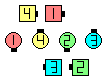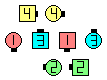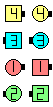Arm Turn 1/4\$B\$N8e(B Centers \$B\$,(B Arm Turn 1/4, Lead End \$B\$,(B Circulate,Trailing End \$B\$,(B 1/2 Circulate\$B\$N8e(B Center Star turns 1/4\$B\$N8e(B Center Wave \$B\$,(B Cast Off 1/4,lonesome Centerdancer \$B\$,(B moves up \$B\$N8e(B (\$B=*\$o\$j(B)

\$BCm
• \$BA4\$F\$N(B Star \$B\$NF0:n\$G\$O(B, 4 \$B?MA40w\$,(B Star \$B\$r:n\$k\$^\$G2s\$k\$N\$rBT\$C\$F\$/\$@\$5\$\$!%(B
• \$B;O\$a\$N(B Arm Turn 1/4 (\$B\$^\$?\$O(B 3/4) \$B\$G(B Lead End \$B\$H\$J\$C\$??M\$O(B full Circulate \$B\$r\$7\$F(B, Center Wave \$B\$N(B End \$B\$H(B Trade \$B\$r\$7\$h\$&\$H\$9\$k\$3\$H\$rHr\$1\$F\$/\$@\$5\$\$!%(B
• \$B1~MQ(B:

• Star 1/4, Star 1/2, Star 3/4
• Don't Turn The Star

{i-j-k-l} 1/4 The Deucey [C4]: \$BJ?9T\$J(B Wave \$B\$+\$i(B: 1/4 The Deucey \$B\$r\$7\$^\$9\$,(B, \$B;O\$a\$N(B 1/4 Turn \$B\$r(B i/4 Turn \$B\$GCV\$-49\$((B, 2 \$BHVL\\$N(B 1/4 Turn \$B\$r(B j/4 Turn \$B\$GCV\$-49\$((B, 3 \$BHVL\\$N(B 1/4 Turn (the Star) \$B\$r(B k/4 Turn \$B\$GCV\$-49\$((B, 4 \$BHVL\\$N(B 1/4 Turn \$B\$r(B l/4 Turn \$B\$GCV\$-49\$(\$^\$9!%J?9T\$J(B Wave \$B\$^\$?\$O(B 2 \$B\$D\$N(B Facing Diamond \$B\$G=*\$o\$j\$^\$9!%(B

Spin Chain The Star [C4] (Charlie Tilley 1969): 2-3-2-3 1/4 The Deucey \$B\$r\$7\$^\$9!%\$b\$7%3!<%i!<\$,(B 'Turn the Star n/4' \$B\$H8@\$C\$?\$H\$-\$O(B, 2-3-n-3 1/4 The Deucey \$B\$r\$7\$^\$9!%(BChoreography for 1/4 (or 3/4) The DeuceyComments? Questions? Suggestions?

https://www.ceder.net/def/14thedeucey.php?language=japan&level=C3A
14-May-2021 19:10:42